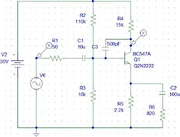133 Pages

## Conceptual DesignEdit

### Functional DescriptionEdit

This block is the preamplifier which takes as an input the small voltage signal generated by a guitar/keyboard and boosts it up to output the same signal at a peak-to-peak swing as high to the rail voltages as possible without distortion. This output can then drive the power amplifier. It is important that this stage introduce as little distortion as possible for high fidelity reproduction at the speaker. The frequency response should extend as far to zero as possible for good low-end response and the high end will be shaped by means of dominant pole compensation which will only attenuate redundant (beyond human hearing) frequencies, whilst ensuring stability for any feedback option we may use.

### Block Diagram and DescriptionEdit

The basic requirements for this functional block are depicted in the diagram. Note that this block diagram may be implemented in a single BJT stage where the voltage amplifier also serves as the input impedance or it may be split into two or more stages where the first stage amplifies the signal only slightly while providing high input impedance, and the second and subsequent stages amplify the signal.

Input Impedance

As most configurations of the BJT as an amplifier act as a voltage controlled current soure, it is important that the input impedance of the amplifier be significantly higher than the output impedance of the source. The input configuration is shown below with the amplifier's input impedance (whatever it may be) is depicted as its Thevenin equivalent and assumed to be purely resistive.

As can be seen, the source will have some impedance modelled by 'Rs' which appears in series with the source voltage and the amplifier input impedance 'Rin'. The voltage 'Vin' serves as the input to the amplifier, hence an AC sinusoid appearing across Rin results in an amplified version of the sinusoid at the amplifier output. We can see that this configuration is a voltage divider as follows:

$V_{in}=V_s.\frac{R_{in}}{R_{in}+R_{s}}$

Hence it is clear that Rin should be large relative to Rs in order to get a large proportion of the voltage appearing across the input to the amplifier. Let us take two examples.

1. Rs = Rin; In this case we only get half our signal at the input of the amplifier, i.e. we have already halved our signal to noise ratio!
2. 50Rs = Rin; In this case we get Vin = 0.98Vs, which is a big improvement.

As demonstrated, it is important to have an amplifier input with a relatively large impedance.

Voltage Amplification

The most important function of this stage of the amplifier is voltage amplification. This means that the input signal from the previous stage (usually < 1V pk-to-pk) should be increase to a much higher level. In order to maximise power delivery from the supply to the speaker, the voltage across the speaker should be something close to the rail voltages although there is a trade off here between distortion and output voltage. BJTS are intrinsically non-linear devices, however if we take a small section of the transfer characteristic (small signal), it becomes very close to linear but when a BJT is outputting a relatively high voltage, non-linearity becomes a problem. A compromise should be reached between acceptable distortion and power output. It is also important that the output impedance be as low as possible for the same reason as described above in "Input Impedance". Think of the output impedance of this stage as Rs and the input impedance of the next stage as Rin.

## Design ParametersEdit

### Input ParametersEdit

 Input Impedance >= 1M.Ohm

### Output ParametersEdit

 Output Impedance < 10kOhm

### Transfer ParametersEdit

 Voltage Gain 16 - 20 THD (Total Harmonic Distortion) < 1% Low Frequency Break Point < 40 Hz High Frequency Break Point 30-40 kHz

## Design ImplementationEdit

The details of on-going design should be maintained here.

### Possible BJT Amp ConfigurationsEdit

• Common-Emitter (with emitter resistance): The ol' standard. Good all-round basic design . What we will probably use.
• Common-Base: Very good high-frequency response, but poor voltage amp characteristics otherwise. Generally used as a current buffer.
• Common-Collector: Poor voltage gain. Low output resistance. Generally used for output stages.
• CC in series with a CE: Good candidate for a two-transistor design. Wider bandwidth than a CE and with a higher input resistance.

??

### Circuit Diagram and DescriptionEdit

Here is a design suggested by me, any criticism or other designs are welcome. Circuit description coming soon! --PeterOliver 10:48, 31 May 2009 (UTC)### AnalysisEdit

#### DC BiasingEdit

At DC, The capacitor C1 and the bypass capacitor C2 are open circuits. If we neglect transistor base current, R2 and R3 form a voltage divider:

$V_B = \frac{10K}{10K+110K}V_{cc} = 2.5V$

If Q1 is in active mode, $V_{BE}$ is 0.7V. So $V_E = V_B-0.7 = 1.8V$.

Now we can work out the biasing currents.

$I_C \approx I_E = \frac{V_E}{R5} = \frac{1.8}{2K} = 0.9 mA$

If $\beta \approx 300$ then we have $I_B = 3\mu A$. Comparing this to the current in the voltage divider,

$\frac{V_{CC}}{R2+R3} = \frac{30}{120K} = 250\mu A \gg 3\mu A$

justifying our neglecting the base current. --SamMay 05:04, 1 June 2009 (UTC)

Coming soon.

#### Feedback AnalysisEdit

Also coming soon.

## Performance TestingEdit

### Input ParametersEdit

 Input Impedance

### Output ParametersEdit

 Output Impedance

### Transfer ParametersEdit

 Voltage Gain 22 THD (Total Harmonic Distortion) <4% at input signal Low Frequency Break Point 8 Hz High Frequency Break Point 24 kHz